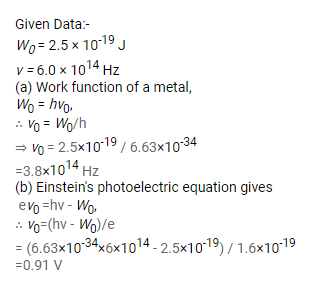# The work function of a metal is

Question:

The work function of a metal is $2.5 \times 10^{-19} \mathrm{~J}$.

(a) Find the threshold frequency for photoelectric emission.

(b) If the metal is exposed to a light beam of frequency $6.0 \times 10^{14} \mathrm{~Hz}$, what will be the stopping potential?

Solution: